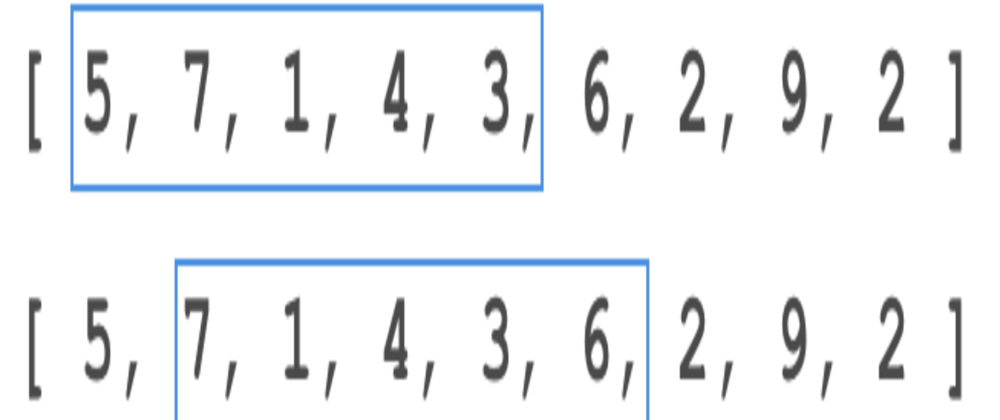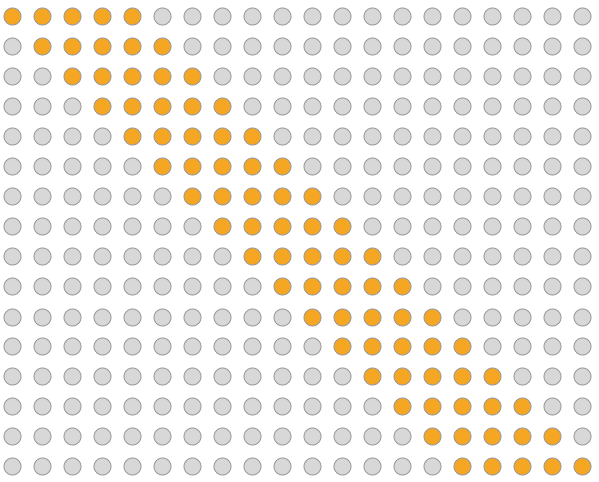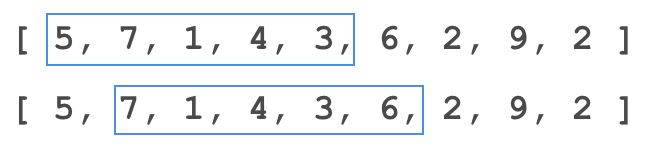## CodeNewbie Community 🌱# Sliding Window Technique

## Introduction

The sliding window technique is used for reducing some redundant calculations that slow down the program. like it can reduce time complexity from O(n^2) to O(n) with O(1) space complexity.

## Why use it?

First of all it reduce time coplexity and also with O(1) space complexity. so let's understand with Example..
So we want to find maximum sum of k consecutive integer from array, so brute force would look like this for k=5,

``````#include<iostream>
#include<vector>
using namespace std;
int main(){
vector<int> arr = {5,2,6,7,7,3,2,1,3,9};
int final_max = 0;
for(int i=0;i<arr.size()-5;i++){
int temp_max = 0;
for(int j=i;j<i+5;j++){
temp_max += arr[j];
}
if(temp_max>final_max){
final_max = temp_max;
}
}
cout << final_max << endl;
return 0;
}
``````

But time complexity of the above program is O(nk)

Brute Force ApproachAs per we can see in the above image brute approach checks every pattern of k length(here k=5). if you compare the above code with this image you will understand it.

here k=5 so it won't make too much difference in O(n) and O(nk) but what if k is too big. then it will impact the running time of the program, so what to do now? can we implement the above code to O(n)?

The answer is YES!! , with the use of the sliding window, we can reduce the time complexity of the above code O(n).

## How does Sliding Window Works?

So let's see how sliding window works.
let me give you simple visual with small array,

``````{5,2,6,7,7,3,2,1,3,9}
for k=3 (because k=5 is too much to write)
{5,2,6} --> first 3 elements
{2,6,7} --> remove 5 and add 7
{6,7,7} --> remove 2 and add 7
{7,7,3} --> remove 6 and add 3
{7,3,2} --> remove 7 and add 2
{3,2,1} --> remove 7 and add 1
{2,1,3} --> remove 3 and add 3
{1,3,9} --> remove 2 and add 9
``````

let's understand with second example,

`````` {5, 7, 1, 4, 3, 6, 2, 9, 2}
k=5
{5,7,1,4,3} --> first 5
{7,1,4,3,6} --> remove 5 and add 6
and so on.
``````As we can see in the above image it moves one step at one time. so this is how actually it works.

## Let's Code

Code for a maximum sum of k consecutive integer from the array, using the sliding window technique.

``````#include<iostream>
#include<vector>
using namespace std;
int sum_of_k_ele(vector<int> arr,int k){
int sum = 0;
for(int i=0;i<k;i++){
sum += arr[i];
}
return sum;
}
int main(){
vector<int> arr = {5,2,6,7,7,3,2,1,3,9};
int k=3;
// below function will be used only once
// for finding sum of first k digits
int final_sum = sum_of_k_ele(arr,k);

int temp_sum = final_sum;
for (int i=k;i<arr.size();i++){
temp_sum = temp_sum - arr[i-k];
temp_sum = temp_sum + arr[i];
if(temp_sum>final_sum){
final_sum = temp_sum;
}
}
cout << final_sum << endl;

return 0;
}
``````

## When to use?

• When you are looking for a subrange in a given string or array-like highest or smallest value or targeted value.
• it involves a data structure that is iterable like a string or array.
• when there can be brute force solution with O(n^2) or (2^n)

## References

Thank You 😊😊